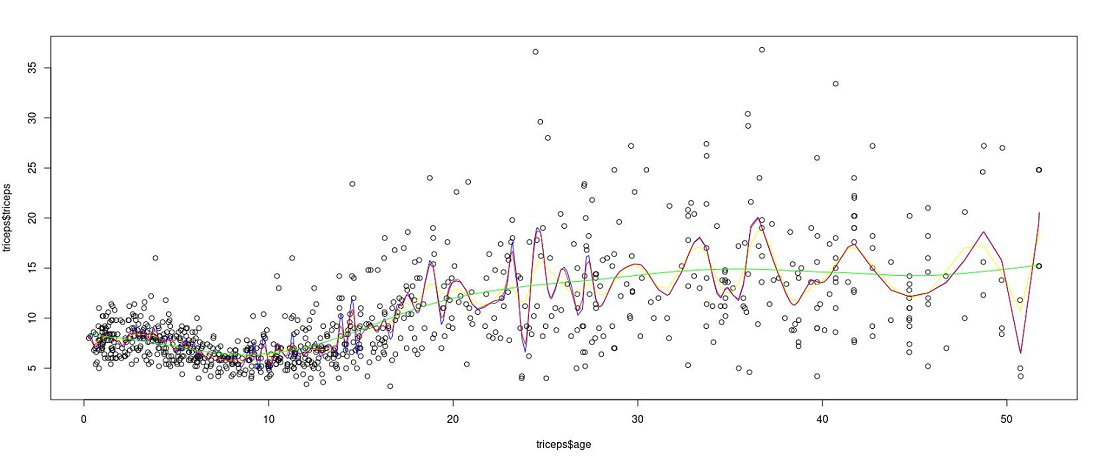GeeksforGeeks App
Open AppBrowser
Continue

# Smoothing spline

A spline curve is a mathematical representation for which it is easy to build an interface that will allow a user to design and control the shape of complex curves and surfaces. The general approach is that the user enters a sequence of points, and a curve is constructed whose shape closely follows this sequence. The points are called control points.

### Cubic Spline:

The cubic spline is a spline that uses the third-degree polynomial which satisfied the given m control points. To derive the solutions for the cubic spline, we assume the second derivation 0 at endpoints, which in turn provides a boundary condition that adds two equations to m-2 equations to make them solvable. The system of equations for the Cubic spline for 1-dimension can be given by:### Interpolating Spline:

In interpolating spline, we need to find the curve that interpolates (xi, yi) such that g(xi) =yi.

### Smoothing Spline:

In the smoothing spline, we will try to fit a spline to the dataset so that we can minimize the Residual by selecting a high degree polynomial for the basis function. We will add a penalization term for the roughness of the fitted curve. That means it as roughness will increase the penalization term also increase in turn increases loss.

The RSS error can be given by:Here, \lambda is the smoothing parameter guiding the trade-off fitting the data and roughness of function. To estimate thewe perform the generalized cross-validation or restricted marginal likelihood.

•No smoothing, the spline converges to interpolating spline.
•Estimate converges to the linear least squares method.

Thus, the λ results in a smooth curve (a straight line in the limit), and a smaller λ leads to a more rough curve. The solution for the above spline that minimizes the above loss is the natural cubic spline with knots at every measured xi.Since xi may have very big values so, it is sufficient in practice to select many knots.

### Existence and Uniqueness of solution:

We first need to get the values of \hat{f}(x_i); i= 1,…,n and from that derive \hat{f}(x) from it

Letbe the vector representing () then, we assume that sum-of-squares part of spline is fixed. Now, we only need to minimizethe minimizer is natural cubic spline that interpolates the points (xi, f^(xi)). The interpolating spline can be written in the form of:And the roughness penalty is given by:Hence, the RSS error term can be given by:Minimum can be achieved by setting \hat{m} = (I + \lambda K)^{-1} Y, where, K is nxn matrix given bywhere, \Delta : (n-2) x n matrix of second difference and W is (n-2) x (n-2) matrix

### Choosing The smoothing parameter:

• There are two methods of performing the smoothing parameter:
• Cross Validation Method: In mathematical terms, the cross-validation method to tune parameteris :where, (m_\lambda)_{ii} represents the ith diagonal element of• Generalized cross-validation:  In the Generalized cross-validation is to replace the denominator 1 – (m_\lambda)_{ii}  in the cross validation to their sum of averages i.e to trace the average:### Implementation:

• In this implementation, we will be implementing the smooth spline with R. We will be using the triceps data that is provided by the MultiKink library. To install this library, we can use install.packages(“”) function in R.

## R

 # Codelibrary(MultiKink) #for triceps datalibrary(ggplot2)   #for the plotsset.seed(2021)     # load trips datadata("triceps")# smooth splinespline0 = smooth.spline(triceps$age,triceps$triceps)# smooth spline with lambda parameterspline1 <- smooth.spline(triceps$age,triceps$triceps, lambda=.000000001) # smooth spline with degree of freedom (equivalent to trace in GCV)spline2 = smooth.spline(triceps$age, triceps$triceps, df =100)# smooth spline with cross-validationspline3= smooth.spline(triceps$age, triceps$triceps, cv =10)  # plot the above dataset with spline linesplot(triceps$age, triceps$triceps)  # plot different splineslines(spline0, col='yellow')lines(spline1, col="blue")lines(spline2, col="red")lines(spline3, col="green")

Output:Plot showing different splines

### References:

My Personal Notes arrow_drop_up